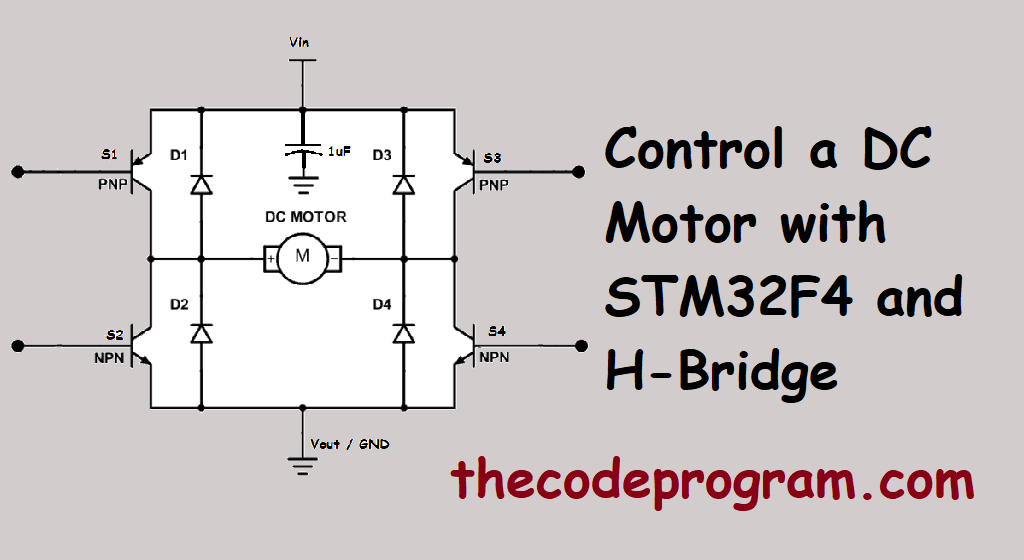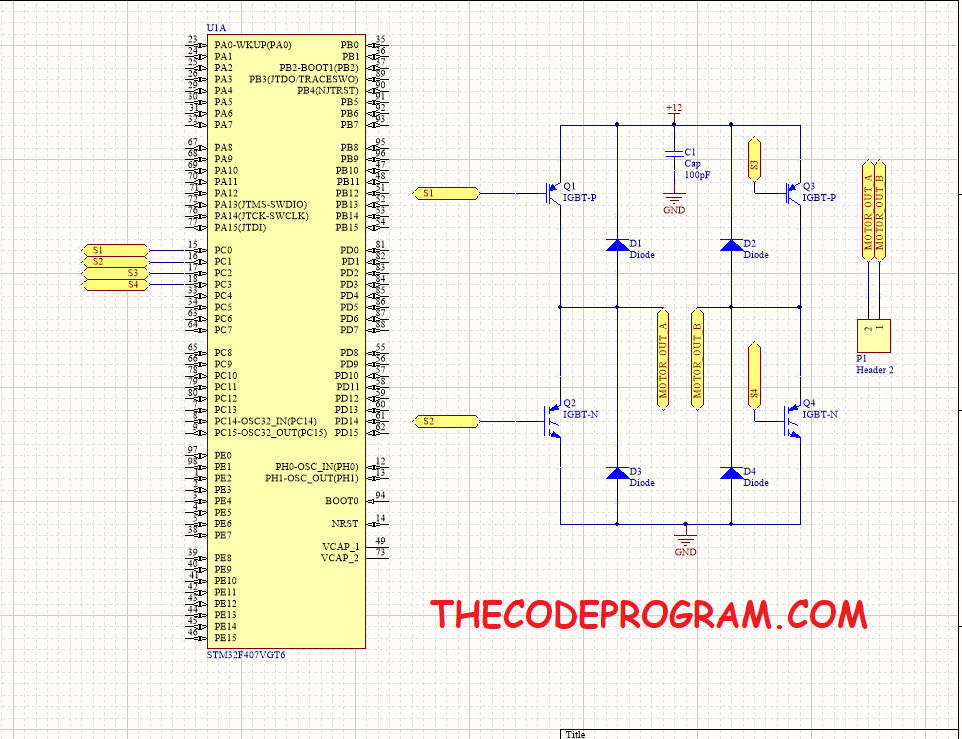01/01/2020 65

## Control a DC Motor with STM32F4 and H-Bridge

Hello everyone, in this article we are going to talk about how can we control a DC motor with H-Bridge and STM32F4 Std Periph library. First we will built a simple schematic on altium designer and after it we are going to write some codes.

Now lets get started then...

I drew a schematic with basic H-Bridge configuration. I will supply big valued current so I have used IGBT instead of BJT transistor. I have located a header with two poles and I will connect the motor inputs to these header. I also located Diodes and a capacitor with ground to protect our circuit.

This schematic is the most simple H-Bridge circuit. I strongly recommend to add additional protection components and circuits. Because you will need to protect your circuit from overloading and overcurrenting or something else problems. So let's keep talking about controlling.

Below image you can see the schematic.As you can see the schematic above we have connected the PC0, PC1, PC2 and PC3 pins to the related inputs on the H-Bridge circuit. I also connected the 12V to supply energy to the circuit.

We will also use two pins to control the direction of the motor rotation. We will connect some push button to the PC4 and PC5 pins.

Create a Keil uVision project and configure pins for controlling the driver switches.

``````
//First enable the clock bus of C port of the STM32F4
RCC_AHB1PeriphClockCmd(RCC_AHB1Periph_GPIOC, ENABLE);
``````

After enabling the related clock bus now we will set the configuration of the related pins.

``````
GPIO_InitTypeDef GPIO_InitStruct;
//Set the pin working mode as output.
GPIO_InitStruct.GPIO_Mode = GPIO_Mode_OUT;
GPIO_InitStruct.GPIO_OType = GPIO_OType_PP;
//Set the required pins
GPIO_InitStruct.GPIO_Pin = GPIO_Pin_0 | GPIO_Pin_1 | GPIO_Pin_2 | GPIO_Pin_3 | GPIO_Pin_4 | GPIO_Pin_5;
GPIO_InitStruct.GPIO_PuPd = GPIO_PuPd_UP;
GPIO_InitStruct.GPIO_Speed = GPIO_Speed_50MHz;
//And initialize the configuration.
GPIO_Init(GPIOC, &GPIO_InitStruct);
``````

After initialization the required pins now we will define two functions. These functions will rotate the motor clockwise and counter clockwise by changing the switching combinations. And our microcontroller decide the rotation directon with the switches.

``````
void fnc_rotateCW(void){
GPIO_ResetBits(GPIOC, GPIO_Pin_0);
GPIO_ResetBits(GPIOC, GPIO_Pin_3);
GPIO_SetBits(GPIOC, GPIO_Pin_1);
GPIO_SetBits(GPIOC, GPIO_Pin_2);
}
``````

And...

``````
void fnc_rotateCCW(void){
GPIO_ResetBits(GPIOC, GPIO_Pin_1);
GPIO_ResetBits(GPIOC, GPIO_Pin_2);
GPIO_SetBits(GPIOC, GPIO_Pin_0);
GPIO_SetBits(GPIOC, GPIO_Pin_3);
}
``````

And do not forget to define prototypes of these functions at the head of the code if you write the functions below the main function. This is important else if your program will not see these functions.

``````
//prototypes of the rotation functions
void fnc_rotateCCW(void);
void fnc_rotateCW(void);

int main(void)
{
GPIO_InitTypeDef GPIO_InitStruct;
//Set the pin working mode as output.
GPIO_InitStruct.GPIO_Mode = GPIO_Mode_OUT;
GPIO_InitStruct.GPIO_OType = GPIO_OType_PP;
//Set the required pins
GPIO_InitStruct.GPIO_Pin = GPIO_Pin_0 | GPIO_Pin_1 | GPIO_Pin_2 | GPIO_Pin_3 | GPIO_Pin_4 | GPIO_Pin_5;
GPIO_InitStruct.GPIO_PuPd = GPIO_PuPd_UP;
GPIO_InitStruct.GPIO_Speed = GPIO_Speed_50MHz;
//And initialize the configuration.
GPIO_Init(GPIOC, &GPIO_InitStruct);

//decide the rotation direction
fnc_rotateCW();
}
fnc_rotateCCW();
}

}
``````

Have a good DC motor controlling.

Burak hamdi TUFAN